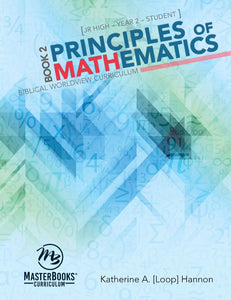# Principles of Mathematics Book 2

Master Books (NLP)

• \$58.59
Unit price per
Shipping calculated at checkout.

Approach Math with Confidence

Mathematical terms and concepts can seem so complex and intimidating as students begin the transition from Jr High to High School math courses€”Katherine Loop€™s down-to-earth style and conversational tone sets students as ease and reminds them to approach new formulas, terms, and concepts with confidence and an understanding that each simply describe a useful tool we can put we can put to use in everyday life, history, science, art, music, and more!

Engaging, Conversational Lessons

Principles of Mathematics Book 2 focuses on pre-algebra concepts and emphasizes practical application, teaching your student both the €˜why€™ and €˜how€™ of math through engaging, conversational lessons. This approach firms up foundational math concepts and prepares students for algebra in a logical, step-by-step way that helps them understand concepts, build problem-solving skills, and see how different aspects of math connect.

Incredibly Faith Building

€œEvery time you solve a math problem, you€™re relying on the underlying consistency present in math. Any time you see that math still operates consistently, it€™s testifying that God is still on His throne, faithfully holding all things together.€ €“ Katherine Loop

In Principles of Mathematics Book 2, each lesson and exercise leads your student towards understanding the character and attributes of God through the study of math history, concepts, and application.

Principles of Mathematics Book 2 goes beyond adding a Bible verse or story to math instruction, it actively teaches and describes how the consistencies and creativity we see in mathematical concepts proclaim the faithful consistency of God Himself and points students towards understanding math through a Biblical worldview.

Excellent High School Preparation

Principles of Mathematics Book 2 lays a solid foundation€”both academically and spiritually€”as your student prepares for high school algebra! Students will study pre-algebra concepts, further develop their problem-solving skills, see how algebraic concepts are applied in a practical way to everyday life, and strengthen their faith!

In Principles of Mathematics Book 2 your student will:

• Explore pre-algebra
• Strengthen critical thinking skills
• Discover why we study math€”and why it matters to each of us
• Transform their view of math through a Biblical Worldview
• Approach mathematics as a tool for exploring & describing God's universe
• Understand algebraic concepts, history, and their practical application
• Fortify their faith
• and so much more!

Principles of Mathematics Book 2 Includes:

• Student Textbook

Course Features:

• 30-45 minutes per lesson, 4-5x per week
• Recommended Grade Level: 7th - 8th

• Preface
• Chapter 1: The Big Picture
• Chapter 2: Mastering Fractions and Negative Numbers
• Chapter 3: Exploring Unknowns and Equations
• Chapter 4: Fractions, Unknowns, and Equations
• Chapter 5: Ratios, Proportions, and Unknowns
• Chapter 6: Percents, Unknowns, and Rearranging Equations
• Chapter 7: Simplifying Expressions
• Chapter 8: Negative Numbers and Unknowns
• Chapter 9: Parentheses, the Distributive Property, and Unknowns
• Chapter 10: Inequalities
• Chapter 11: Statistics
• Chapter 12: Probability
• Chapter 13: Exploring Mathematical Relationships
• Chapter 14: Graphing Mathematical Relationships
• Chapter 15: Exponents and Unknowns
• Chapter 16: More with Exponents
• Chapter 17: Square Roots and Unknowns
• Chapter 18: Glimpses of Trigonometry
• Chapter 19: Sets, Sequences, Symbols, and Unknowns
• Chapter 20: Glimpses of Consumer Math
• Chapter 21: Review
• Appendix A: Reference
• Appendix B: Mathematical Relationships: Graphs and Functions
• Endnotes
• Index